GRE Subject Test: Math : Matrix Operations

Example Questions

Example Question #1 : Linear Algebra

Perform the following operation.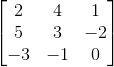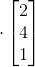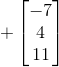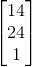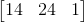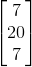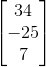Explanation:

The first step to solving this operation is to do the multiplication: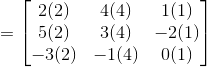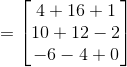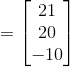Once we have multiplied the matrices, we can perform the addition portion: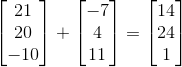Example Question #1 : Matrix Operations

Perform the following operation.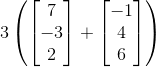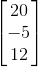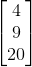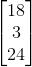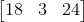Explanation:

The first step is to solve whatever is in the parentheses, in this case it is addition: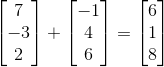We then substitute our solution into the parentheses: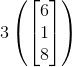Our next, and final step in this problem, is to carry out the multiplication: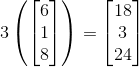All GRE Subject Test: Math Resources